# Uniform algebra

(diff) ← Older revision | Latest revision (diff) | Newer revision → (diff)

A subalgebra, closed with respect to the topology of uniform convergence, of the algebraof continuous functions on a compactumthat contains all constant functions and separates the points of. The last condition means that for each pairof distinct points inthere is a functioninfor which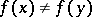. Uniform algebras are usually provided with the sup norm: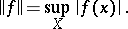Here. Every Banach algebra with an identity (even without assuming commutativity) and with a norm satisfying the latter condition is isomorphic to a uniform algebra.

The uniform algebras form an important subclass of the class of commutative Banach algebras (cf. Commutative Banach algebra) over the field of complex numbers.

To each pointcorresponds a homomorphism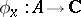, defined by. Thereforeis in a natural way topologically imbedded in the space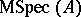of maximal ideals of, and under the corresponding identificationcontains the Shilov boundary (cf. Boundary (in the theory of uniform algebras)). In the study of uniform algebras a major role is played by peak points (that is, points ofat which the strict maximum modulus of at least one element ofis attained), by multiplicative probability measures on(that is, representing measures of homomorphisms fromto) and by measures onthat are orthogonal to. Many concrete results relating to uniform algebras touch on the relations between these notions.

A uniform algebra is called symmetric if with each function its complex conjugate belongs to the algebra. According to the Stone–Weierstrass theorem, each symmetric uniform algebra on a compactumcoincides with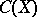. The so-called anti-symmetric uniform algebras, containing no real-valued functions apart from the constants, form a kind of opposite class. A typical example is the algebra of all functions that are analytic in the open unit disc of the complex plane and continuous on its closure (the disc algebra). The Shilov–Bishop theorem: Each uniform algebra can be obtained from anti-symmetric uniform algebras by "glueing" in a certain way. Even more refined classification theorems are known. At the same time arbitrary uniform algebras do not reduce to algebras of analytic functions of the type of the disc algebra. For example, it is possible to construct a uniform algebra on a one-dimensional compactum, which coincides with its space of maximal ideals, such that all points of the compactum are peak points and at the same time only the zero element of the algebra can be zero on a non-empty open set.

How to Cite This Entry:
Uniform algebra. Encyclopedia of Mathematics. URL: http://encyclopediaofmath.org/index.php?title=Uniform_algebra&oldid=13486
This article was adapted from an original article by E.A. Gorin (originator), which appeared in Encyclopedia of Mathematics - ISBN 1402006098. See original article